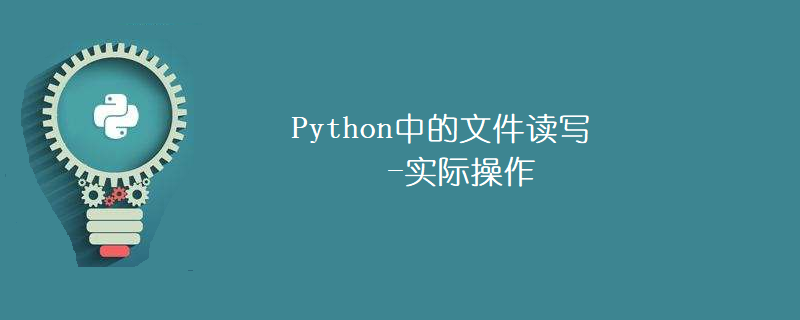Jquery中文网 www.jquerycn.cn
Jquery中文网 >  Python编程  >  Python入门  >  正文 Python中的文件读写-实际操作

# Python中的文件读写-实际操作

jquery中文网为您提供Python中的文件读写-实际操作等资源，欢迎您收藏本站，我们将为您提供最新的Python中的文件读写-实际操作资源

Python中的文件读写-实际操作file_object = open(filename, mode)

open函数中的模式告诉Python你要对文件做什么。处理文本文件时可以指定多种模式。

'w'- 写入模式：当需要更改文件并更改或添加信息时，使用此模式。请记住，这会擦除现有文件以创建新文件。文件指针位于文件的开头。

'r'- 读取模式：当文件中的信息仅用于读取而不以任何方式更改时，使用此模式。文件指针位于文件的开头。

'a'- 附加模式：此模式自动将信息添加到文件末尾。文件指针放在文件的末尾。

'r '- 读/写模式：当您要更改文件并从中读取信息时使用。文件指针位于文件的开头。

'a '- 附加和读取模式：打开文件以允许将数据添加到文件末尾，并让程序也读取信息。文件指针放在文件的末尾。

'x'- 独占创建模式：此模式专门用于创建文件。如果已存在同名文件，则函数调用将失败。

data_file = open("workData.txt", "r ")

`data_file.close（）`

```>>> f = open("/tmp/myfile.txt", "w")
>>> f.close()
>>> f.read()Traceback (most recent call last):
File "<input>", line 1, in <module>
f.read()ValueError: I/O operation on closed file.```

```with open("workData.txt", "r ") as workData:
# File object is now open.
# Do stuff with the file:
# File object is now closed.
# Do other things...```

```This data is on line 1
This data is on line 2
This data is on line 3```

```with open("workData.txt", "r ") as work_data:
print("This is the file name: ", work_data.name)
print(line)```

```This is the file name: workData.txt
This data is on line 1
This data is on line 2
This data is on line 3```

`line = workData.read(6)`

```This is the file name: workData.txt
This d```

```with open("workData.txt", "r ") as work_data:
print("This is the file name: ", work_data.name)
print(line_data)```

```This is the file name:  workData.txt
This data is on line 1```

`print(work_data.readlines())`

`['This data is on line 1', 'This data is on line 2', 'This data is on line 3']`

```with open("workData.txt", "r ") as work_data:
for line in work_data:
print(line)```

```This data is on line 1
This data is on line 2
This data is on line 3```

`work_data.write("This data is on line 4\n")`

```values = [1234, 5678, 9012]with open("workData.txt", "a ") as work_data:
for value in values:
str_value = str(value)
work_data.write(str_value)
work_data.write("\n")```

0 - 表示文件的开头

1 - 表示当前指针位置

2 - 表示文件的结尾

```s data is on line 1
This data is on line 2
This data is on line 3```

```with open("workData.txt", "a ") as work_data:
print(work_data.tell())```

```# Open the file as read-only
with open("workData.txt", "r") as work_data:
work_data_contents.insert(1, "This goes between line 1 and 2\n")
# Re-open in write-only format to overwrite old file
with open("workData.txt", "w") as work_data:
work_dataContents = "".join(work_data_contents)
work_data.write(work_data_contents)```

```with open("workData.txt", "r") as work_data:
for line in work_data:
print(line)```

```This data is on line 1
This goes between line 1 and 2
This data is on line 2
This data is on line 3```

[]